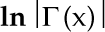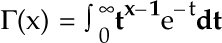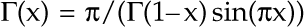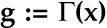JavaScript is required to for searching.
 Skip Navigation Links Exit Print Viewman pages section 3: Extended Library Functions

### , gammal_r

- log gamma function

### Synopsis

```c99 [ flag... ] file... -lm [ library... ]
#include <math.h>

extern int signgam;

double lgamma(double x);```
`float lgammaf(float x);`
`long double lgammal(long double x);`
`double gamma(double x);`
`float gammaf(float x);`
`long double gammal(long double x);`
`double lgamma_r(double x, int *signgamp);`
`float lgammaf_r(float x, int *signgamp);`
`long double lgammal_r(long double x, int *signgamp);`
`double gamma_r(double x, int *signgamp);`
`float gammaf_r(float x, int *signgamp);`
`long double gammal_r(long double x, int *signgamp);`

### Description

These functions returnwherefor x > 0 andfor x < 1.

These functions use the external integer signgam to return the sign of |~(x) while lgamma_r() and gamma_r() use the user-allocated space addressed by signgamp.

### Return Values

Upon successful completion, these functions return the logarithmic gamma of x.

If x is a non-positive integer, a pole error occurs and these functions return +HUGE_VAL, +HUGE_VALF, and +HUGE_VALL, respectively.

If x is NaN, a NaN is returned.

If x is 1 or 2, +0 shall be returned.

If x is ±Inf, +Inf is returned.

### Errors

These functions will fail if:

Pole Error

The x argument is a negative integer or 0.

If the integer expression (math_errhandling & MATH_ERREXCEPT) is non-zero, then the divide-by-zero floating-point exception is raised.

### Usage

An application wanting to check for exceptions should call feclearexcept(FE_ALL_EXCEPT) before calling these functions. On return, if fetestexcept(FE_INVALID | FE_DIVBYZERO | FE_OVERFLOW | FE_UNDERFLOW) is non-zero, an exception has been raised. An application should either examine the return value or check the floating point exception flags to detect exceptions.

In the case of lgamma(), do not use the expression signgam*exp(lgamma(x)) to computeInstead compute lgamma() first:

lg = lgamma(x); g = signgam*exp(lg);

only after lgamma() has returned can signgam be correct. Note that |~(x) must overflow when x is large enough, underflow when -x is large enough, and generate a division by 0 exception at the singularities x a nonpositive integer.

### Attributes

See attributes(5) for descriptions of the following attributes:

ATTRIBUTE TYPE
ATTRIBUTE VALUE
Interface Stability
See below.
MT-Level
See below.

The lgamma(), lgammaf(), lgammal(), and gamma() functions are Standard. The lgamma_r(), lgammaf_r(), lgammal_r(), gamma_r(), gammaf_r(), and gammal_r(), functions are Stable.

The lgamma(), lgammaf(), lgammal(), gamma(), gammaf(), and gammal() functions are Unsafe in multithreaded applications. The lgamma_r(), lgammaf_r(), lgammal_r(), gamma_r(), gammaf_r(), and gammal_r() functions are MT-Safe and should be used instead.

 Copyright © 2010, Oracle and/or its affiliates. All rights reserved. Legal Notices